# Math 113 Problem Set 3

Due October 3, 2003

1. Show that your favorite trigonometric identity continues to hold for complex values of the arguments. (You might, for instance, show that the usual angle-addition formulas for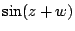and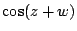continue to hold for complex values of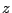and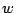.)
2. Bak and Newman, chapter 3, p. 41, problem 11. (An entire'' function is a complex differentiable function that is defined on all of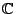.)
3. Bak and Newman, chapter 3, p. 42, problem 17.
4. Bak and Newman, chapter 3, p. 42, problem 18. (This was mentioned but not proved in class. Make sure you understand what this implies for the image of vertical or horizontal lines.)
5. Needham, Chapter 2, pp. 113-114, problem 12.
6. Needham, Chapter 2, pp. 114-115, problem 15.
7. Consider the mapping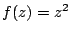.
1. Find the images of horizontal and vertical lines under this mapping.
2. Show directly that the image curves intersect at right angles, and thus that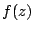maps little squares to little rectangles. (Of course, we have shown already that the map is conformal, but try to show this directly.)
3. (optional) Find geometrically the image of an arbitrary line. Show that the map is conformal when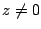by showing that the angle between two arbitrary lines is preserved by the mapping.
8. We saw in class that the exponential function maps vertical straight lines to circles centered at the origin. The goal of this problem is to see to what extent the converse is true: what are all the conformal functions which map vertical lines to circles?
1. Consider the map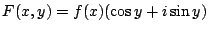, for a real-valued function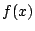. Show that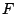maps vertical lines to circles. Find all such functionsso thatis a conformal map.
2. What happens ifis complex-valued? Do you get any more solutions?
3. (optional) Extend the argument above to find all complex differentiable functions onwhich map vertical lines to circles.